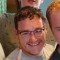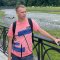# xPDOQuery.whereLast updated Dec 22nd, 2019 | Page history | Improve this page | Report an issue

## xPDOQuery::where¶

Add a WHERE condition to the query. In general, the way this works is you append an operator to an attribute after a colon.

``````array('attribute:operator' => 'value')
``````
Operator Symbol Example
Equals none `\$query->where(array('width' => 15));`
Not Equals != `\$query->where(array('width:!=' => 15));`
Greater Than > `\$query->where(array('width:>' => 15));`
Less Than < `\$query->where(array('width:<' => 15));`
Greater Than or Equal to >= `\$query->where(array('width:>=' => 15));`
Less Than or Equal to <= `\$query->where(array('width:<=' => 15));`
Like LIKE `\$query->where(array('width:LIKE' => '%15%'));`
Not Like NOT LIKE `\$query->where(array('width:NOT LIKE' => '%15%'));`
Exists in IN `\$query->where(array('width:IN' => array(15,16,17,20)));`
Not Exists in NOT IN `\$query->where(array('width:NOT IN' => array(15,16,17,20)));`
Is Null IS `\$query->where(array('width:IS' => null));`

## Syntax¶

API Docs: xPDOQuery::where()

``````xPDOQuery where ([mixed \$conditions = ''],
[string \$conjunction = xPDOQuery::SQL_AND],
[mixed \$binding = null],
[integer \$condGroup = 0])
``````

## Examples¶

Get all Boxes with width of 15.

``````\$query = \$xpdo->newQuery('Box');
\$query->where(array(
'width' => 15,
));
\$boxes = \$xpdo->getCollection('Box',\$query);
``````

Get all boxes with a width of 15 or 10.

``````\$query = \$xpdo->newQuery('Box');
\$query->where(array('width' => 15));
\$query->where(array('width' => 10),xPDOQuery::SQL_OR); // you can use orCondition here as well
\$boxes = \$xpdo->getCollection('Box',\$query);
``````

An alternative method to get boxes with a width of 15 or 10.

``````\$query = \$xpdo->newQuery('Box');
\$query->where(array(
array( // two arrays used to contain the OR statement within the listed conditions
'width' => 15
),
array(
'width' => 10
)
),xPDOQuery::SQL_OR); // use one array if no additional where statements are used.
\$boxes = \$xpdo->getCollection('Box',\$query);
``````

Next alternative method to get boxes with a width of 15 or 10.

``````\$query = \$xpdo->newQuery('Box');
\$query->where(array(
array(
'width' => 15
),
array(
'OR:width:=' => 10
)
));
\$boxes = \$xpdo->getCollection('Box',\$query);
``````

Grab all boxes with a width greater than or equal to 15, but not with a width of 23.

``````\$query = \$xpdo->newQuery('Box');
\$query->where(array(
'width:>=' => 15,
'width:!=' => 23,
));
\$boxes = \$xpdo->getCollection('Box',\$query);
``````

Get all boxes with a name with the letter 'q' in it:

``````\$query = \$xpdo->newQuery('Box');
\$query->where(array(
'name:LIKE' => '%q%',
));
\$boxes = \$xpdo->getCollection('Box',\$query);
``````

Using and & or in the same query to get all boxes with a width of 15 or 10 and a height between 10 and 15.

``````\$query = \$xpdo->newQuery('Box');
\$query->where(array(
array(
'width:=' => 15, // note that adding 'AND:' or 'OR:' in front of the attribute, an operator must be used ':='
'OR:width:=' => 10
),
array(
'AND:height:>=' => 10,
'AND:height:<=' => 15
)
));
\$boxes = \$xpdo->getCollection('Box',\$query);
``````

### More Complex Examples¶

If you have a more complex model with many joined tables, the where method should refer only to the class alias (not the class name) that contains the attribute. Below is an example of a query passed to the getCollectionGraph method, where you can see that myTable object is joined through to the user profile information.

``````\$query = \$modx->newQuery('myTable');
\$query->where(array('Profile.fullname:LIKE' => '%Company%'));
\$records = \$this->ParentCMS->getCollectionGraph('myTable', '{"modUser": {"Profile":{} } }',\$query);
``````

Another method is to pass a \$criteria array immediately as the 2nd argument to newQuery. Notice how the alias "Resource" is used since that's what is listed as the alias in the schema definition for the modTemplateVarResource object:

``````\$criteria = array();
\$criteria['modTemplateVarResource.tmplvarid'] = 9;
\$criteria['modTemplateVarResource.value:IN'] = array('Red','Green','Blue');
\$criteria['Resource.template'] = 2;
\$criteria = \$modx->newQuery('modTemplateVarResource', \$criteria);
\$tvrs = \$modx->getCollectionGraph('modTemplateVarResource','{"Resource":{}}', \$criteria);
``````

Here are a couple of different examples of doing subqueries: